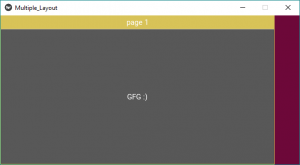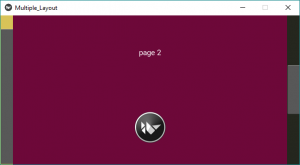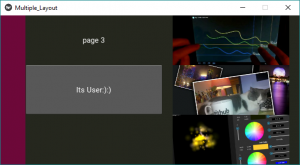Open in App
Not now

# Python | Layouts in layouts (Multiple Layouts) in Kivy

• Last Updated : 22 Sep, 2021

Kivy is a platform independent GUI tool in Python. As it can be run on Android, IOS, linux and Windows etc. It is basically used to develop the Android application, but it does not mean that it can not be used on Desktops applications.
In this article, we are going to discuss how we can use layouts in layouts basically multiple Layouts the most basic example of it.
Most of the time we are able to use one layout but having difficulty to use multiple layouts.

In kivy there are many Types of Layouts:

1. AnchorLayout: Widgets can be anchored to the ‘top’, ‘bottom’, ‘left’, ‘right’ or ‘center’.
2. BoxLayout: Widgets are arranged sequentially, in either a ‘vertical’ or a ‘horizontal’ orientation.
3. FloatLayout: Widgets are essentially unrestricted.
4. RelativeLayout: Child widgets are positioned relative to the layout.
5. GridLayout: Widgets are arranged in a grid defined by the rows and cols properties.
6. PageLayout: Used to create simple multi-page layouts, in a way that allows easy flipping from one page to another using borders.
7. ScatterLayout: Widgets are positioned similarly to a RelativeLayout, but they can be translated, rotate and scaled.
8. StackLayout: Widgets are stacked in a lr-tb (left to right then top to bottom) or tb-lr order.

Note:You can use as many as you can in a single file.

Basic Approach to create multiple layouts in one file:

```1) import kivy
2) import kivyApp
3) import BoxLayout
4) import
4) set minimum version(optional)
5) Extend the container class
6) set up .kv file :
7) create App class
8) return container class or layout
9) Run an instance of the class```

Implementation of the Approach –
main.py file

Note:
If you were using .kv file to make multiple layouts There is no need to import Gridlayout, Boxlayout, AnchorLayout, FloatLayout, StackLayout, PageLayout, Button, etc. As .kv file supports all this as It has all this imported already. But if doing this without .kv file you must have to import these.

## Python3

 `## Sample Python application demonstrating the ` `## Program of How to use Multiple Layouts in Single file `   `########################################################################`   `# import kivy module   ` `import` `kivy ` `  `  `# base Class of your App inherits from the App class.   ` `# app:always refers to the instance of your application  ` `from` `kivy.app ``import` `App`   `# this restrict the kivy version i.e ` `# below this kivy version you cannot ` `# use the app or software ` `kivy.require(``'1.9.0'``)`   `# creates the button in kivy  ` `# if not imported shows the error` `from` `kivy.uix.button ``import` `Button ` `  `  `# BoxLayout arranges children in a vertical or horizontal box. ` `# or help to put the childrens at the desired location. ` `from` `kivy.uix.boxlayout ``import` `BoxLayout`   `# The GridLayout arranges children in a matrix.` `# It takes the available space and` `# divides it into columns and rows,` `# then adds widgets to the resulting “cells”.` `from` `kivy.uix.gridlayout ``import` `GridLayout`   `# The PageLayout class is used to create` `# a simple multi-page layout,` `# in a way that allows easy flipping from` `# one page to another using borders.` `from` `kivy.uix.pagelayout ``import` `PageLayout`     `########################################################################`   `# creating the root widget used in .kv file ` `class` `MultipleLayout(PageLayout):` `    ``pass`   `########################################################################`   `# creating the App class in which name ` `#.kv file is to be named PageLayout.kv ` `class` `Multiple_LayoutApp(App): ` `    ``# defining build() ` `    ``def` `build(``self``): ` `        ``# returning the instance of root class ` `        ``return` `MultipleLayout() `   `########################################################################` `    `  `# creating object of Multiple_LayoutApp() class` `MlApp ``=` `Multiple_LayoutApp()`   `# run the class` `MlApp.run()`

In this file we are creating/using all the layout as it is Simpler to built/ use all these in .kv file
.kv file of the code

## Python3

 `# Program of How to use Multiple Layouts in Single .kv file`   `########################################################################`   `# creating page Layout` `:`   `#########################################################################` `    `  `    ``# Creating Page 1` `    `  `    ``# Using BoxLayout inside PageLayout` `    ``BoxLayout:`   `        ``# creating Canvas` `        ``canvas:` `            ``Color:` `                ``rgba: ``216` `/` `255.``, ``195` `/` `255.``, ``88` `/` `255.``, ``1` `            ``Rectangle:` `                ``pos: ``self``.pos` `                ``size: ``self``.size`   `        ``# Providing orientation to the BoxLayout` `        ``orientation: ``'vertical'`   `        ``# Adding Label to Page 1` `        ``Label:` `            ``size_hint_y: ``None` `            ``height: ``1.5` `*` `self``.texture_size[``1``]` `            ``text: ``'page 1'`   `        ``# Creating Button` `        ``Button:` `            ``text: ``'GFG :)'` `            `  `            ``# Adding On_press function` `            ``# i.e binding function to press / touch` `            ``on_press: ``print``(``"This Is The First Page"``)`   `#########################################################################`   `    ``# Creating Page 2`   `    ``BoxLayout:` `        ``orientation: ``'vertical'` `        ``canvas:` `            ``Color:` `                ``rgba: ``109` `/` `255.``, ``8` `/` `255.``, ``57` `/` `255.``, ``1` `            ``Rectangle:` `                ``pos: ``self``.pos` `                ``size: ``self``.size` `        ``Label:` `            ``text: ``'page 2'`   `        ``# This Image is directly from the websource` `        ``# By using AsyncImage you can use that` `        ``AsyncImage:` `            ``source: ``'http://kivy.org / logos / kivy-logo-black-64.png'`   `##########################################################################`   `    ``# Creating Page 3`   `    ``# Using The Second Layout` `    ``# Creating GridLayout` `    ``GridLayout:`     `        ``canvas:` `            ``Color:` `                ``rgba: ``37` `/` `255.``, ``39` `/` `255.``, ``30` `/` `255.``, ``1` `            ``Rectangle:` `                ``pos: ``self``.pos` `                ``size: ``self``.size`     `        ``# Adding grids to Page 3` `        ``# It may be row or column` `        ``cols: ``2`     `        ``# In first Grid` `        ``# Adding Label + Image` `        ``Label:` `            ``text: ``'page 3'`   `        ``AsyncImage:` `            ``source: ``'http://kivy.org/slides/kivyandroid-thumb.jpg'`     `        ``# In Second Grid` `        ``# Adding Button + Image` `        ``Button:` `            ``text: ``'Its User:):)'` `            ``on_press: ``print``(``"Heloo User This is the Last Page"``)`   `        ``AsyncImage:` `            ``source: ``'http://kivy.org/slides/kivypictures-thumb.jpg'`     `        ``# In third grid` `        ``# Adding Widget + Image` `        `  `        ``Widget`   `        ``AsyncImage:` `            ``source: ``'http://kivy.org/slides/particlepanda-thumb.jpg'`

Output:
Image 1:Image 2:Image 3:Video Output:

My Personal Notes arrow_drop_up
Related Articles